alonsoformula In Spanish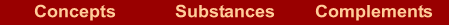What are they?  |  How are they named? If we know the formula  | If we know the name  |  Examples  |  Exercises

What are they?

They are ternary compounds containing a metallic element, and many groups OH-(hydroxide) as the oxidation number of the metal. They are ionic compounds formed by metal cations and anions OH-.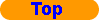How are they named?

Stock´s nomenclature: it is formed by two words. The first word is the name of the metal, followed immediately by the oxidation number between brackets and in Roman numbers. If the oxidation number of the metal is invariable, it is not indicated. The second word is "hydroxide"

METAL(N) hydroxideIf we know the formula

In the formula: the oxidation number of the metal is equal to the number of ions OH.If we know the name

In the name: Remember that you must know the symbols of the elements and the oxidation numbers which are invariable.

1. Write the symbol of the first element.
2. Write the group OH.
3. The number of OH matches up with the oxidation number of metal.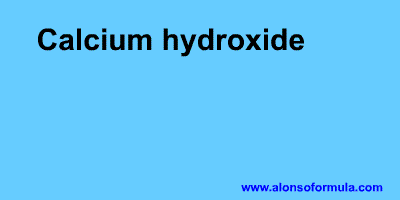Examples

 LiOH Lithium hydroxide Ba(OH)2 Barium hydroxide Fe(OH)3 Iron(III) hydroxide Cr(OH)3 Chromium(III) hydroxide Al(OH)3 Aluminium hydroxideExercises

In Formulae you have an exercise to write the names of these substances and to check your results. You also have the answer to the exercise.

In Names you have an exercise to write the formulae for these substances and to check your results. You have to introduce the formulae without subscripts, for example for water = H2O. You also have the answer to the exercise.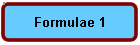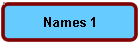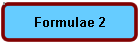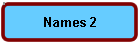Home | Introduction | Oxidation number | Mechanics | Kind of substances | Elementary substances | Metal oxides | Nonmetal oxides | Metal-nonmetal compounds | Nonmetal-nonmetal compounds | Metal hydrides | Hydracids | H with nonmetal | Hydroxides | Oxyacids | Oxysalts | Acid salts | Exercises | Periodic Table | Links
Author: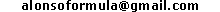WWW.ALONSOFORMULA.COM Formulación InorgánicaFormulación OrgánicaFormulación Inorgánica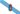Formulación OrgánicaFormulació Inorgánica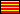Formulació OrgánicaEzorganikoaren Formulazioa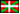Nomenclature of Inorganic Q.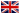Física y Química de ESOFísica e Química de ESOFQ de 1º de BachilleratoFQ de 1º de BacharelatoQuímica de 2º de BachilleratoPrácticas de Química